# Voltage

Voltage is electric potential energy per unit charge, measured in joules per coulomb ( = volts). It is often referred to as "electric potential", which then must be distinguished from electric potential energy by noting that the "potential" is a "per-unit-charge" quantity. Like mechanical potential energy, the zero of potential can be chosen at any point, so the difference in voltage is the quantity which is physically meaningful. The difference in voltage measured when moving from point A to point B is equal to the work which would have to be done, per unit charge, against the electric field to move the charge from A to B. When a voltage is generated, it is sometimes called an "electromotive force" or emf.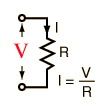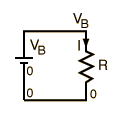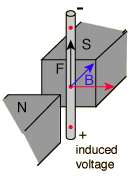Used to calculate current in Ohm's law. Used to express conservation of energy around a circuit in the voltage law. Used to calculate the potential from a distribution of charges. Is generated by moving a wire in a magnetic field.
 Measurement with voltmeter
 Analogy with pressure in water circuit
Index

Voltage concepts

 HyperPhysics*****Electricity and magnetism R Nave
Go Back

# Electromotive Force (EMF)

When a voltage is generated by a battery, or by the magnetic force according to Faraday's Law, this generated voltage has been traditionally called an "electromotive force" or emf. The emf represents energy per unit charge (voltage) which has been made available by the generating mechanism and is not a "force". The term emf is retained for historical reasons. It is useful to distinguish voltages which are generated from the voltage changes which occur in a circuit as a result of energy dissipation, e.g., in a resistor.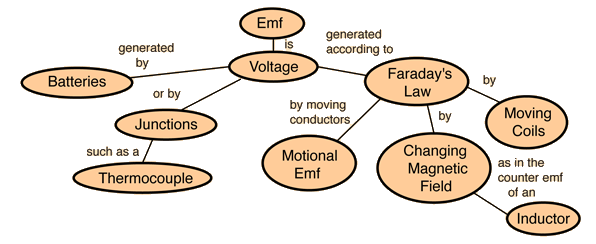Index

Voltage concepts

 HyperPhysics*****Electricity and magnetism R Nave
Go Back

# Motional EMF

The magnetic force exerted on the charges in a moving conductor will generate a voltage (a motional emf). The generated voltage can be seen to be the work done per unit charge. This motional emf is one of many settings in which the generated emf is described by Faraday's Law.Note that the direction of the magnetic force is shown as the right hand rule direction on a positive charge, and shows the direction of the conventional current in the loop.

Index

Voltage concepts

 HyperPhysics*****Electricity and magnetism R Nave
Go Back

# Motional EMF and Faraday's LawThe motional emf expression is an application of Faraday's Law, as can be seen from: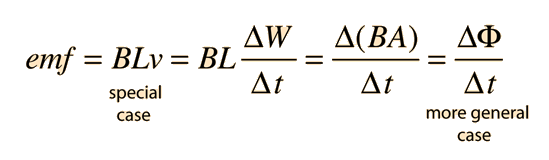Index

Voltage concepts

 HyperPhysics*****Electricity and magnetism R Nave
Go Back